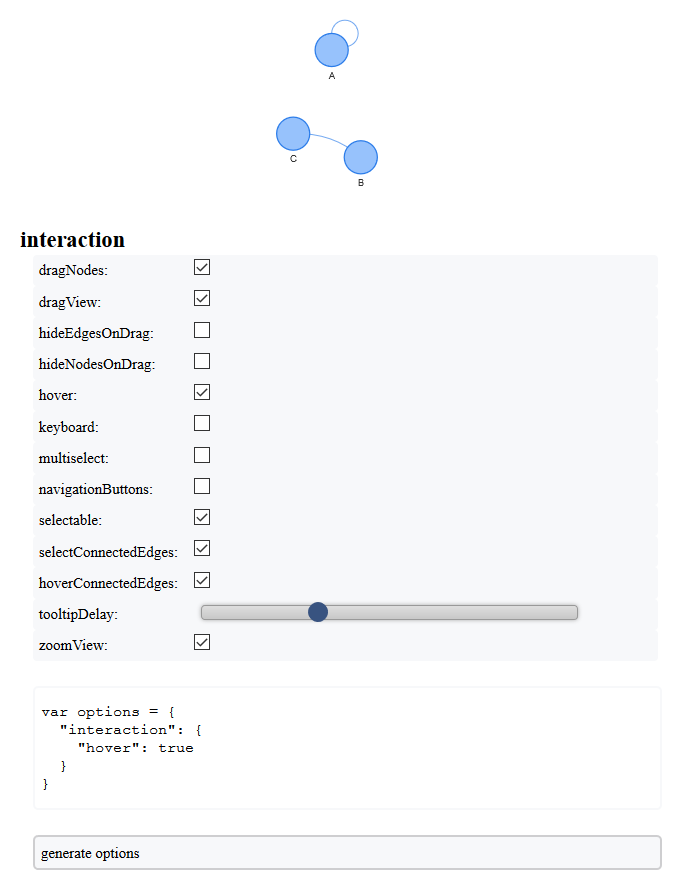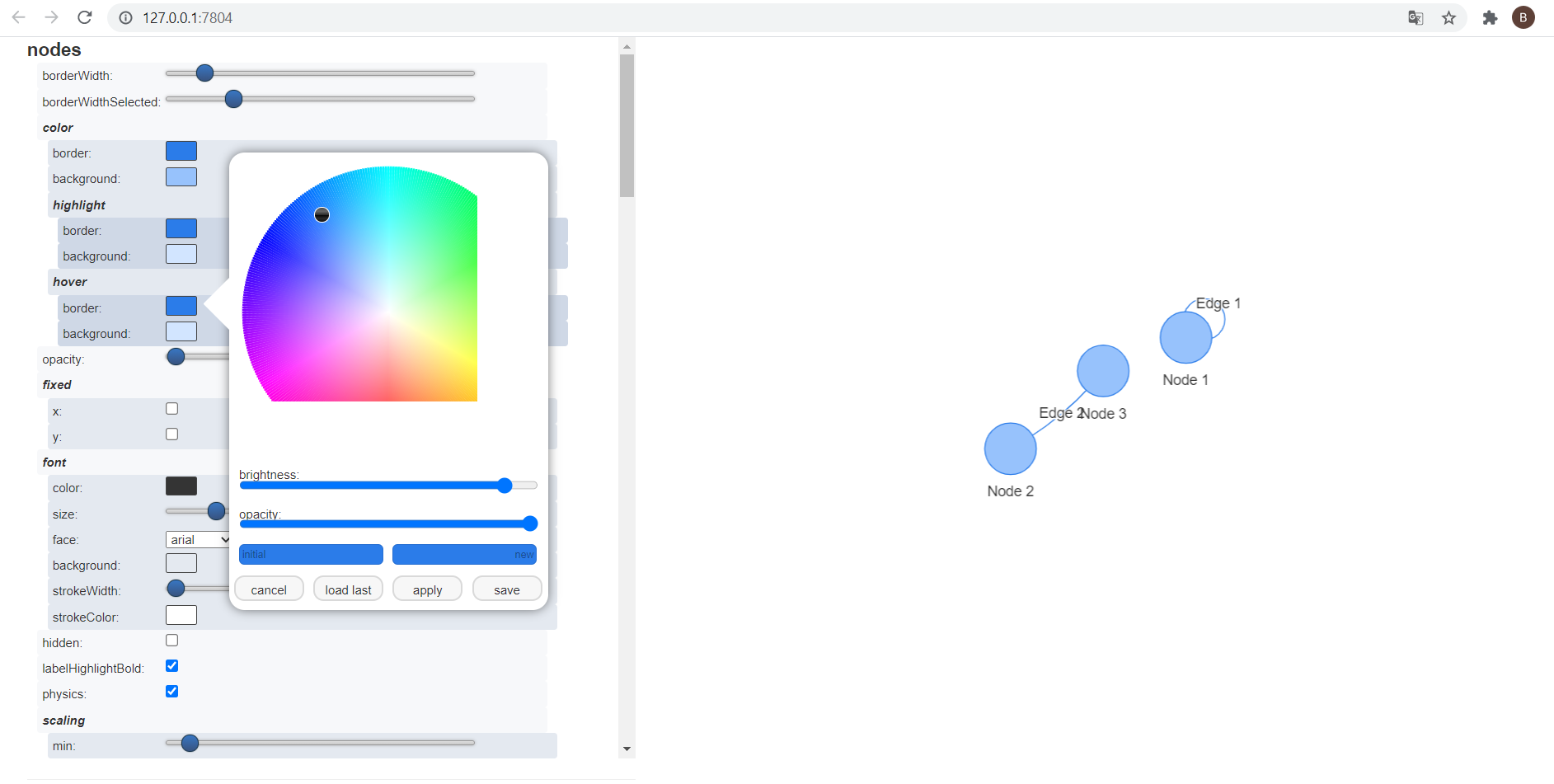## Use visConfigure() to configure and view options directly on your network

Don’t show here. Test and open the network in a web browser. Can be very usefull to configure your network as wanted !

nodes <- data.frame(id = 1:3, label = LETTERS[1:3])
edges <- data.frame(from = c(1,2), to = c(1,3))

# don't look in RStudio viewer
visNetwork(nodes, edges, width = "100%") %>%
visConfigure(enabled = TRUE)## or prefer the shiny app / module visNetworkEditor !

nodes <- data.frame(id = 1:3, label = paste("Node", 1:3))
edges <- data.frame(from = c(1,2), to = c(1,3), label = paste("Edge", 1:2))
network <- visNetwork(nodes, edges)

custom_network <- visNetworkEditor(object = network)
custom_network